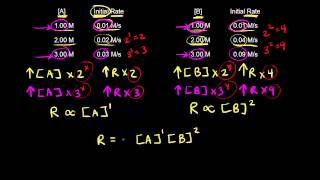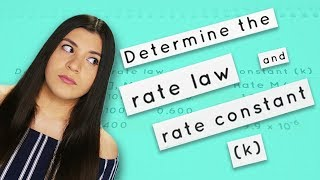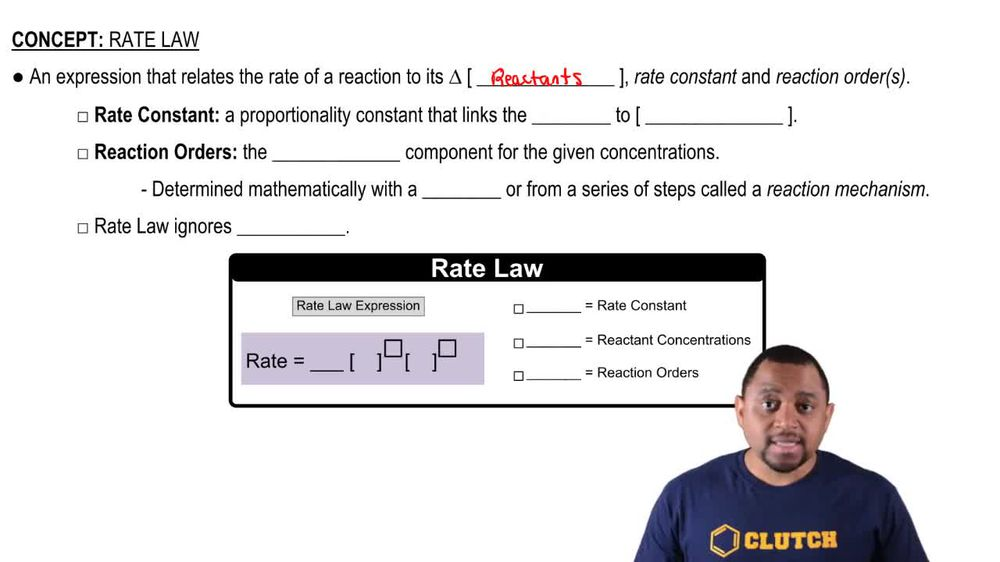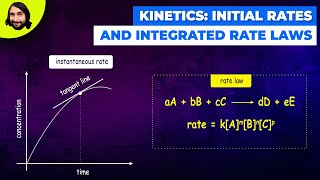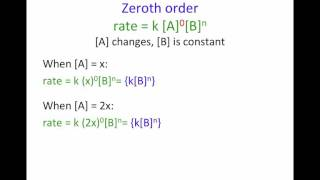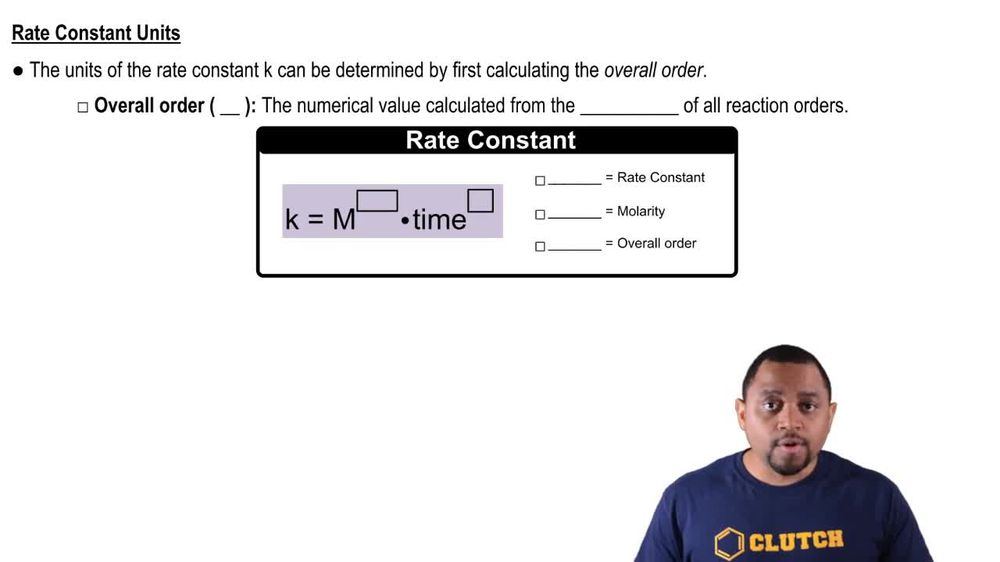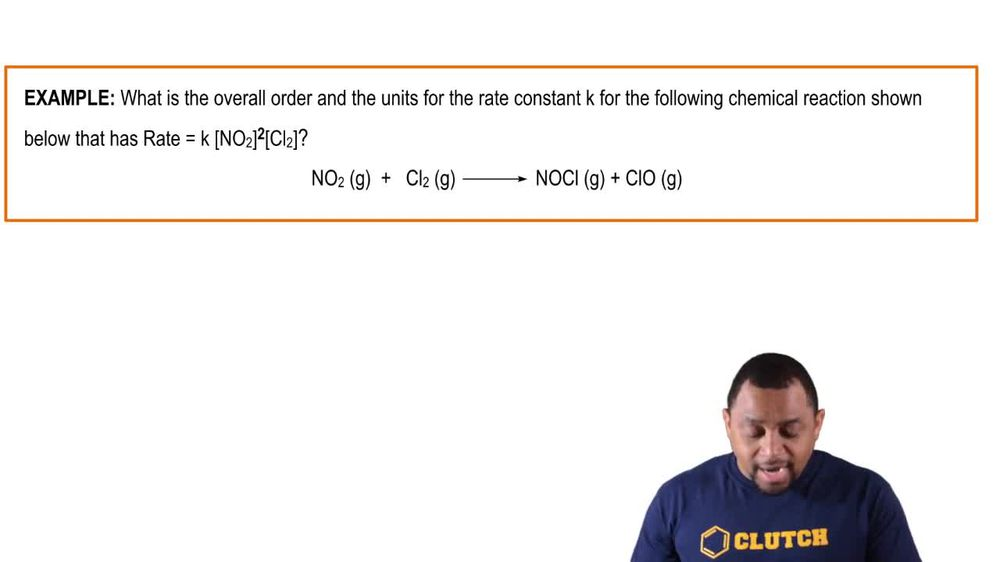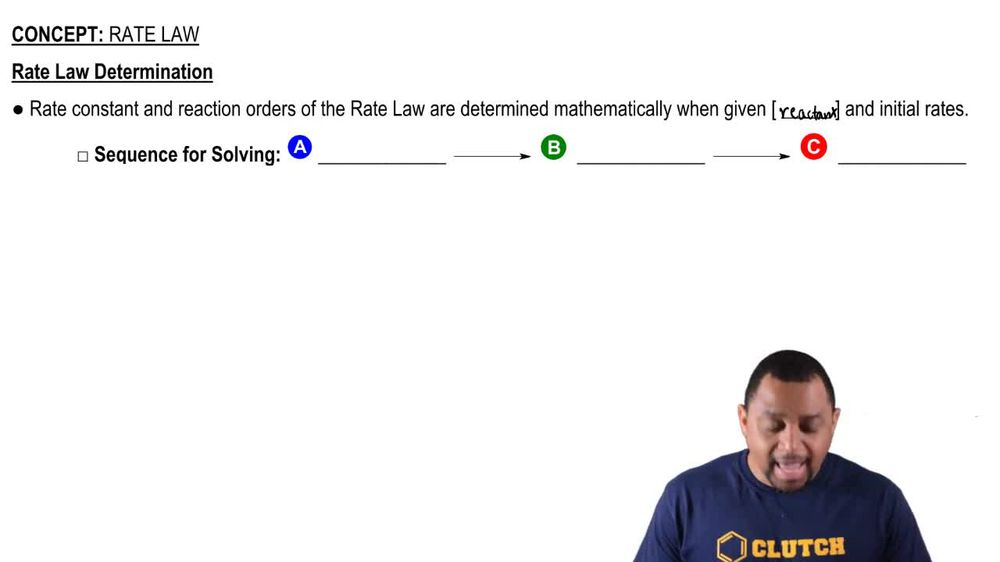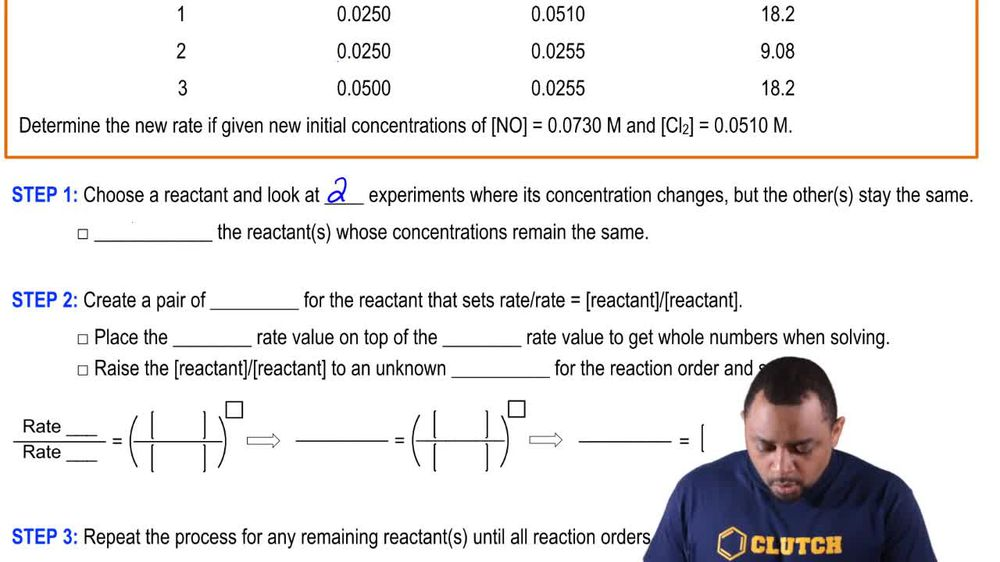Start typing, then use the up and down arrows to select an option from the list.
1. 15. Chemical Kinetics2. Rate Law# Rate Law Concept 2

by Jules Bruno
107 views
1
0
Now the units for the rate constant K can be determined by first calculating the overall order. Now our overall order uses the variable end. It's a numerical value calculated from the addition of all reaction orders. And here the formula to figure out the units for your rate constant K is K equals M two and plus one times time inverse. So remember Kesari constant, capital M is your polarity, and and again is your overall order. So adding up all the orders of your rate law helps you to figure out and take that and plug it into this formula and you'll know what the units for K will be.